Algebra Commons™

1,047 Full-Text Articles 1,224 Authors 224,226 Downloads118 Institutions

All Articles in Algebra

1,047 full-text articles. Page 4 of 39.

2019University of Colorado, Boulder

Induction Of Nontrivial Supercharacter Theories For Finite Groups, Jesse Franklin

This study focuses on the partitions of a group that arise from: action by conjugation, a two sided multiplicative generalization of conjugation, and inclusion of a subgroup into the group. Since conjugacy classes correspond to irreducible characters, studying the partitions in a group compatible with conjugacy classes in the subgroup, and by analogy, studying the partition of a group compatible with superclasses in a subgroup, invariances in the group can be derived from the subgroup's simpler structure. The fusion of conjugacy classes, and superclasses, has some effects on the calculation of an induced and superinduced function. However, these effects ...

2019University of Colorado, Boulder

Visualizing Random Walks On Supercharacter Theories For Uppertriangular Unipotent Matrices, Justin Willson

The conjugacy classes of some finite groups are provably wild in the sense that there is no general solution to finding their conjugacy classes other than brute force. One solution to this problem is to create a supercharacter theory for a group which has the effect of gluing together conjugacy classes into more manageable chunks. In this paper we apply these methods to the group of uppertriangular unipotent matrices and analyze the combinatorics of this added structure.

2019University of Colorado, Boulder

Induction Of Nontrivial Supercharacter Theories For Finite Groups, Jesse Franklin

This study focuses on the partitions of a group that arise from: action by conjugation, a two sided multiplicative generalization of conjugation, and inclusion of a subgroup into the group. Since conjugacy classes correspond to irreducible characters, studying the partitions in a group compatible with conjugacy classes in the subgroup, and by analogy, studying the partition of a group compatible with superclasses in a subgroup, invariances in the group can be derived from the subgroup's simpler structure. The fusion of conjugacy classes, and superclasses, has some effects on the calculation of an induced and superinduced function. However, these effects ...

Sharp Bounds For Decomposing Graphs Into Edges And Triangles, 2019Iowa State University

Sharp Bounds For Decomposing Graphs Into Edges And Triangles, Adam Blumenthal, Bernard Lidicky, Oleg Pikhurko, Yanitsa Pehova, Florian Pfender, Jan Volec

Mathematics Conference Papers, Posters and Presentations

Let pi3(G) be the minimum of twice the number of edges plus three times the number of triangles over all edge-decompositions of G into copies of K2 and K3. We are interested in the value of pi3(n), the maximum of pi3(G) over graphs G with n vertices. This specific extremal function was first studied by Gyori and Tuza [Decompositions of graphs into complete subgraphs of given order, Studia Sci. Math. Hungar. 22 (1987), 315--320], who showed that pi3(n)<9n2/16.
In a recent advance on this problem, Kral, Lidicky, Martins and Pehova [arXiv:1710:08486] proved via flag ...

A Study Of Entanglement Theory, 2019Iowa State University

A Study Of Entanglement Theory, Thang Tran

Creative Components

This article is a 50-page study of entanglement theory. First, the basic of quantum mechanics and mathematical definition of entanglement is presented. Second, entanglement is described as a physical phenomenon that set Quantum Mechanics distinct from Classical Physics. Third, entanglement as a physical resource for quantum communication is discussed with applications, the distillation process, and quantification problem. Fourth, several methods to detect entanglement are addressed. Detecting entanglement is shown to have a closed connection with positive maps in operator theory.

A (Co)Algebraic Approach To Hennessy-Milner Theorems For Weakly Expressive Logics, 2019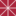Université de Lorraine

A (Co)Algebraic Approach To Hennessy-Milner Theorems For Weakly Expressive Logics, Zeinab Bakhtiari, Helle Hvid Hansen, Alexander Kurz

Engineering Faculty Articles and Research

"Coalgebraic modal logic, as in [9, 6], is a framework in which modal logics for specifying coalgebras can be developed parametric in the signature of the modal language and the coalgebra type functor T. Given a base logic (usually classical propositional logic), modalities are interpreted via so-called predicate liftings for the functor T. These are natural transformations that turn a predicate over the state space X into a predicate over TX. Given that T-coalgebras come with general notions of T-bisimilarity  and behavioral equivalence , coalgebraic modal logics are designed to respect those. In particular, if two states are behaviourally ...

2019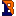Bucknell University

Positivity Among P-Partition Generating Functions Of Partially Ordered Sets, Nate Lesnevich

Honors Theses

We find necessary and separate sufficient conditions for the difference between two labeled partially ordered set's (poset) partition generating functions to be positive in the fundamental basis. We define the notion of a jump sequence for a poset and show how different conditions on the jump sequences of two posets are necessary for those posets to have an order relation in the fundamental basis. Our sufficient conditions are of two types. First, we show how manipulating a poset's Hasse diagram produces a poset that is greater according to the fundamental basis. Secondly, we also provide tools to explain ...

Factorization Lengths In Numerical Monoids, 2019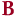Bard College

Factorization Lengths In Numerical Monoids, Maya Samantha Schwartz

Senior Projects Spring 2019

A numerical monoid M generated by the natural numbers n_1, ..., n_k is a subset of {0, 1, 2, ...} whose elements are non-negative linear combinations of the generators n_1, ..., n_k. The set of factorizations of an element in M is the set of all the different ways to write that element as a linear combination of the generators. The length of a factorization of an element is the sum of the coefficients of that factorization. Since an element in a monoid can be written in different ways in terms of the generators, its set of factorization lengths may contain more than ...

Totally Acyclic Complexes, 2019Georgia Southern University

Totally Acyclic Complexes, Holly M. Zolt

Electronic Theses and Dissertations

We consider the following question: when is every exact complex of injective modules a totally acyclic one? It is known, for example, that over a commutative Noetherian ring of finite Krull dimension this condition is equivalent with the ring being Iwanaga-Gorenstein. We give equivalent characterizations of the condition that every exact complex of injective modules (over arbitrary rings) is totally acyclic. We also give a dual result giving equivalent characterizations of the condition that every exact complex of flat modules is F-totally acyclic over an arbitrary ring.

2019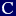Colby College

Basis Reduction In Lattice Cryptography, Raj Kane

Honors Theses

We develop an understanding of lattices and their use in cryptography. We examine how reducing lattice bases can yield solutions to the Shortest Vector Problem and the Closest Vector Problem.

2019Bard College

The Conditional Probability That An Elliptic Curve Has A Rational Subgroup Of Order 5 Or 7, Meagan Kenney

Senior Projects Spring 2019

Let E be an elliptic curve over the rationals. There are two different ways in which the set of rational points on E can be said to be divisible by a prime p. We will call one of these types of divisibility local and the other global. Global divisibility will imply local divisibility; however, the converse is not guaranteed. In this project we focus on the cases where p=5 and p=7 to determine the probability that E has global divisibility by p, given that E has local divisibility by p.

2019University of Denver

Decidability For Residuated Lattices And Substructural Logics, Gavin St. John

Electronic Theses and Dissertations

We present a number of results related to the decidability and undecidability of various varieties of residuated lattices and their corresponding substructural logics. The context of this analysis is the extension of residuated lattices by various simple equations, dually, the extension of substructural logics by simple structural rules, with the aim of classifying simple equations by the decidability properties shared by their extensions. We also prove a number of relationships among simple extensions by showing the equational theory of their idempotent semiring reducts coincides with simple extensions of idempotent semirings. On the decidability front, we develop both semantical and syntactical ...

Counting And Coloring Sudoku Graphs, 2019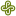Portland State University

Counting And Coloring Sudoku Graphs, Kyle Oddson

Mathematics and Statistics Dissertations and Theses

A sudoku puzzle is most commonly a 9 × 9 grid of 3 × 3 boxes wherein the puzzle player writes the numbers 1 - 9 with no repetition in any row, column, or box. We generalize the notion of the n2 × n2 sudoku grid for all n ϵ Z ≥2 and codify the empty sudoku board as a graph. In the main section of this paper we prove that sudoku boards and sudoku graphs exist for all such n we prove the equivalence of 's construction using unions and products of graphs to the definition of the sudoku graph ...

Homological Constructions Over A Ring Of Characteristic 2, 2019Georgia Southern University

Homological Constructions Over A Ring Of Characteristic 2, Michael S. Nelson

Electronic Theses and Dissertations

We study various homological constructions over a ring $R$ of characteristic $2$. We construct chain complexes over a field $K$ of characteristic $2$ using polynomials rings and partial derivatives. We also provide a link from the homology of these chain complexes to the simplicial homology of simplicial complexes. We end by showing how to construct all finitely-generated commutative differential graded $R$-algebras using polynomial rings and partial derivatives.

Positive Subreducts In Finitely Generated Varieties Of Mv-Algebras, 2019Chapman University

Positive Subreducts In Finitely Generated Varieties Of Mv-Algebras, Leonardo M. Cabrer, Peter Jipsen, Tomáš Kroupa

Mathematics, Physics, and Computer Science Faculty Articles and Research

Positive MV-algebras are negation-free and implication-free subreducts of MV-algebras. In this contribution we show that a finite axiomatic basis exists for the quasivariety of positive MV-algebras coming from any finitely generated variety of MV-algebras.

Active Learning In Computer-Based College Algebra, 2019Portland State University

Active Learning In Computer-Based College Algebra, Steven Boyce, Joyce O'Halloran

Mathematics and Statistics Faculty Publications and Presentations

We describe the process of adjusting the balance between computerbased learning and peer interaction in a college algebra course. In our first experimental class, students used the adaptive-learning program ALEKS within an emporium-style format. Comparing student performance in the emporium format class with that in a traditional lecture format class, we found an improvement in procedural skills, but a weakness in the students’ conceptual understanding of mathematical ideas. Consequently, we shifted to a blended format, cutting back on the number of ALEKS (procedural) topics and integrating activities that fostered student discourse about mathematics concepts. In our third iteration using ALEKS ...

Riemann-Hilbert Problem, Integrability And Reductions, 2019Institute of Mathematics and Informatics, Bulgarian Academy of Sciences

Riemann-Hilbert Problem, Integrability And Reductions, Vladimir Gerdjikov, Rossen Ivanov, Aleksander Stefanov

Articles

Abstract. The present paper is dedicated to integrable models with Mikhailov reduction groups GR ≃ Dh. Their Lax representation allows us to prove, that their solution is equivalent to solving Riemann-Hilbert problems, whose contours depend on the realization of the GR-action on the spectral parameter. Two new examples of Nonlinear Evolution Equations (NLEE) with Dh symmetries are presented.

Gershgorin Type Sets For Eigenvalues Of Matrix Polynomials, 2018National Technical University of Athens

Gershgorin Type Sets For Eigenvalues Of Matrix Polynomials, Christina Michailidou, Panayiotis Psarrakos

Electronic Journal of Linear Algebra

New localization results for polynomial eigenvalue problems are obtained, by extending the notions of the Gershgorin set, the generalized Gershgorin set, the Brauer set and the Dashnic-Zusmanovich set to the case of matrix polynomials.

Regularity Radius: Properties, Approximation And A Not A Priori Exponential Algorithm, 2018Charles University, Faculty of Mathematics and Physics, Department of Applied Mathematics, Prague, Czech Republic and Institute of Computer Science, Czech Academy of Sciences, Prague, Czech Republic.

Regularity Radius: Properties, Approximation And A Not A Priori Exponential Algorithm, David Hartman, Milan Hladik

Electronic Journal of Linear Algebra

The radius of regularity, sometimes spelled as the radius of nonsingularity, is a measure providing the distance of a given matrix to the nearest singular one. Despite its possible application strength this measure is still far from being handled in an efficient way also due to findings of Poljak and Rohn providing proof that checking this property is NP-hard for a general matrix. There are basically two approaches to handle this situation. Firstly, approximation algorithms are applied and secondly, tighter bounds for radius of regularity are considered. Improvements of both approaches have been recently shown by Hartman and Hlad\'{i ...

Commutators Involving Matrix Functions, 2018P.h.D student

Commutators Involving Matrix Functions, Osman Kan, Süleyman Solak

Electronic Journal of Linear Algebra

Some results are obtained for matrix commutators involving matrix exponentials $\left(\left[e^{A},B\right],\left[e^{A},e^{B}\right]\right)$ and their norms.